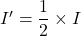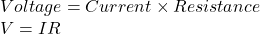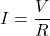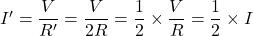## An electric circuit consists of a variable resistor connected to a source of constant potential difference. If the resistance of the resist

Question

An electric circuit consists of a variable resistor connected to a source of constant potential difference. If the resistance of the resistor is doubled, the current through the resistor is: a) halved doubled b) quartered c) quadrupled.

in progress 0
5 months 2021-08-31T05:28:32+00:00 1 Answers 2 views 0

The correct option is a) halved

Therefore,

Current through the resistor will be halved i.eExplanation:

Given:

V = Voltage potential Difference constant

R’ = 2R

To Find:

Current, I’ = ? when R’ = 2R

Solution:

Ohm’s law:

Ohm’s law states that the current through a conductor between two points is directly proportional to the voltage across the two points.

Ohm’s law given asSubstituting the R’ = 2R , new current we getTherefore,

Current through the resistor will be halved i.e